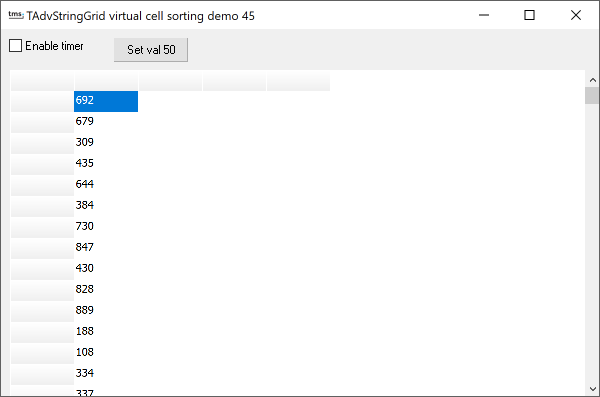### Example 45 : virtual cell sortingThis example combines the techniques of using virtual cell as discussed in example 41 with sort independent cell access shown in example 40.

In this sample, the values shown in the grid column 1 are not stored in the grid cells itself but in an array of integer values declared as:

```values : array[0..100] of
integer;
```

The normal way for using this array of values as virtual cell content for column 1 would be:

```procedure TForm1.AdvStringGrid1GetDisplText(Sender: TObject; ACol,
ARow: Integer; var Value: String);
begin
if (ACol = 1) and (ARow > 0) then
Value := InttoStr(Values[ARow]);
end;
```

Note that with this approach, the grid would always display values in visual row 1, values in visual row 2 etc ... We do want though that the grid display can be sorted (without resorting to sorting the values array as well) This can be achieved by using the sort-independent row access as discussed in example 40 and thus becomes:

```procedure TForm1.AdvStringGrid1GetDisplText(Sender: TObject; ACol,
ARow: Integer; var Value: String);
begin
if (ACol = 1) and (ARow > 0) then
end;
```

Note that it is required to initialize the sort independent access with AdvStringGrid1.InitSortXRef before allowing the user to sort the grid by clicking the column header.

While this approach is working fine, the sample takes this a step further. Suppose that due to an external cause a value in the values array changes (for example due to a measurement doing a real-time update of a value) In this case, the grid does not know the value has been changed and at the same time the application does not know which cell corresponds with the values as the user could have sorted the grid in any way he wanted. Again the unsorted cell access helps. When the value ARow changes in the values array, the grid can thus be updated with:

```values[arow] := newvalue;